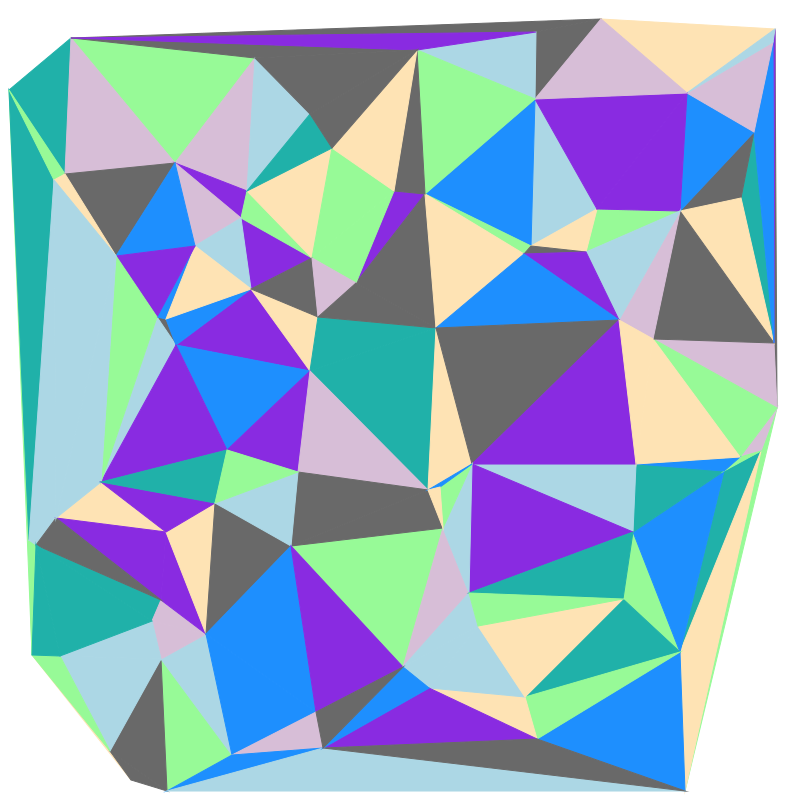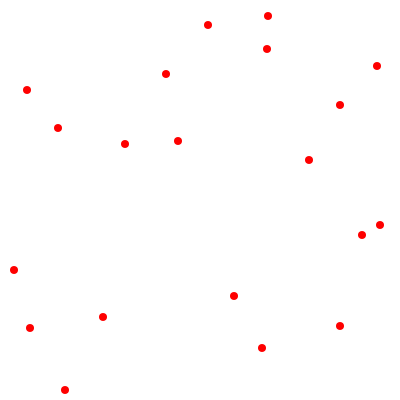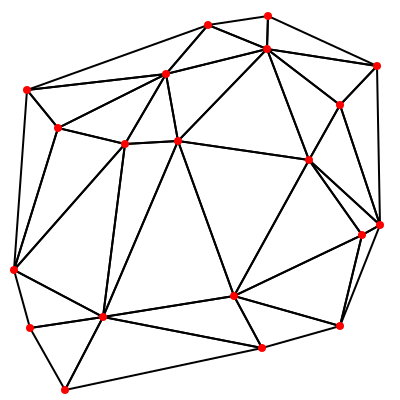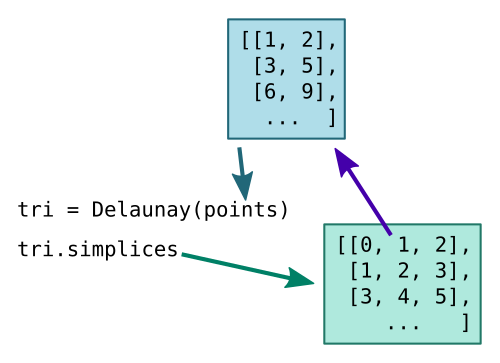# Delaunay triangulation with scipy and generativepy

Martin McBride
2019-06-16

In this post we will see how to perform Delaunay triangulation in Python, using scipy and generativepy.

We will create an image like this:## Delaunay triangulation

You may have heard of Delaunay triangulation, but what is it? Put simply, if you have a set of points like this:Delaunay triangulation joins the dots to create a set of triangles, like this:There are obviously many different ways to do this. The Delaunay method is a particular way of creating triangles so that:

• None of the lines cross.
• The triangles tend to be as close as possible to equalilateral. Delaunay avoids too many long pointy triangles, although it isn't always possible to avoid them altogether.

In the simple example we are looking at, the points are picked at random, and our main aim is to create a pretty image. But there are other uses for Delaunay triangulation. It can be used to find optimal routes on a network, or for sub-dividing shapes in 3d modelling, or for distorting images in complex ways - for example "face swap" images - among other things. In those cases, the points are often chosen carefully, rather than randomly as we are doing, but the technique is identical.

## Delaunay triangulation in scipy

scipy is a Python library containing lots of different numerical functions. Fortunately, many of the functions can be used standalone. One such function is Delaunay in the scipy.spatial module.

This function inputs a list of (x, y) points, and outputs a list of corresponding triangles using the Delaunay algorithm. The diagram below shows more details:• The Delaunay function accepts a list points containing a list of [x, y] points.
• The result is a triangles object tri.
• The simplices field of tri contains a list of triangles.
• Each triangle in the list contains 3 integers which are indexes into the original points list. These give the 3 corners of the triangle.

## Drawing the image in generativepy

To use the Delaunay function in generativepy, you first need to install scipy. See scipy.org for details.

You will need to import the function:

from scipy.spatial import Delaunay


In our draw function we will create a list of random points, using a list comprehension. This just creates a set of points where x and y are random values between 0 and 799:

    SIZE = 800
points = [(random.randrange(SIZE), random.randrange(SIZE))
for i in range(80)]


Next we call the Delaunay function, then loop over the resulting tri.simplices. Each time through the loop, a, b and c are the indexes of the corners of the current triangle. When we call the triangle function, we look up indexes a, b and c in the original points list.

    tri = Delaunay(points)
for a, b, c in tri.simplices:
triangle(canvas, points[a], points[b], points[c])


The triangle function draws each triangle. We pick a random colour for each triangle from the colors list (see the full code below):

def triangle(canvas, a, b, c):
color = random.choice(colors)
canvas.fill(color)
canvas.stroke(color)
canvas.strokeWeight(1)
canvas.triangle(*a, *b, *c)


## Full code

Here is the complete code:

from generativepy import drawing
from generativepy.drawing import Color, ROUND
from scipy.spatial import Delaunay
import random

SIZE = 800

colors = [
Color('palegreen'),
Color('lightblue'),
Color('thistle'),
Color('moccasin'),
Color('lightseagreen'),
Color('dodgerblue'),
Color('blueviolet'),
Color('dimgray'),
]

def triangle(canvas, a, b, c):
color = random.choice(colors)
canvas.fill(color)
canvas.stroke(color)
canvas.strokeWeight(1)
canvas.triangle(*a, *b, *c)

def draw(canvas):
random.seed(40)
points = [(random.randrange(SIZE), random.randrange(SIZE))
for i in range(80)]

tri = Delaunay(points)
for a, b, c in tri.simplices:
triangle(canvas, points[a], points[b], points[c])

drawing.makeImage("delaunay-random-color.png", draw, pixelSize=(SIZE, SIZE),
background=Color(1, 1, 1))